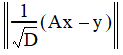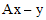# NMath User's Guide

21.4 Weighted Least Squares (.NET, C#, CSharp, VB, Visual Basic, F#)

NMath provides class for solving weighted least squares (WLS) problems. WLS can modulate the importance of each observation in the final solution to correct for violations of the homoscedasticity assumption in ordinary least squares, to give less weight to outliers, or to give less weight to observations thought to be less reliable.

DoubleCOWeightedLeastSq uses a complete orthogonal decomposition technique.1 The computed solution minimizes the 2-norm of the weighted residual vectorwhere D is a diagonal weight matrix whose diagonal consists of the weights.

Prerequisites on the matrix A are that it has more rows than columns, and is of full rank. Note that the algorithm satisfies an accuracy bound that is not affected by ill conditioning in the weight matrix D.

Instances of DoubleCOWeightedLeastSq are constructed from a matrix of observations and a vector of weights. For example:

Code Example – C# weighted least squares

```var A = new DoubleMatrix( "5x2[1 2  1 3  1 6  1 10  1 7]" );
```
```var weights = new DoubleVector( A.Rows, .2, .2 );
```
```var wls = new DoubleCOWeightedLeastSq( A, weights );

```

Code Example – VB weighted least squares

```Dim A As New DoubleMatrix("5x2[1 2  1 3  1 6  1 10  1 7]")
```
```Dim Weights As New DoubleVector(A.Rows, 0.2, 0.2)
```
```Dim WLS As New DoubleCOWeightedLeastSq(A, Weights)
```

In this case, the weights are arbitrary—observations are simply given increasingly higher weights.

DoubleCOWeightedLeastSq provides a Solve() method that accepts a vector y, and computes the solution:

Code Example – C# weighted least squares

```var y = new DoubleVector( "[3 6 8 10 11]" );
```
```var solution = wls.Solve( y );
```

Code Example – VB weighted least squares

```Dim Y As New DoubleVector("[3 6 8 10 11]")
```
```Dim Solution As DoubleVector = WLS.Solve(Y)
```

Other properties and methods on DoubleCOWeightedLeastSq include:

Property A gets the original matrix of observations.

ResidualVector() returns the residual vector.

ResidualNormSqr() computes the 2-norm squared of the residual vector.

Factor() factors other matrices.

Reweight() updates the weights.

NMath provides a selection of weighting functions for use in iteratively reweighted least squares (IRLS; Section 21.5). These functions can also be used to create weights for WLS. Typical weighting functions used in IRLS are a function of the adjusted residuals from the previous iteration. For example, this code computes an ordinary least squares solution, then uses the resulting residuals to solve the same problem using WLS, downweighting the outliers:

Code Example – C# weighted least squares

```// compute ordinary least squares solution
```
```var ols = new DoubleQRLeastSq( A );
```
```DoubleVector olsSolution = ols.Solve( y );
```
```DoubleVector olsResiduals = ols.ResidualVector( y );

```

```// compute weights from residuals using bisquare function
```
```var weights = new DoubleVector( residuals.Length );
```
```IDoubleLeastSqWeightingFunction weightingFunction =
```
```  new DoubleBisquareWeightingFunction( A );
```
```weightingFunction.GetWeights( olsResiduals, ref weights );

```

```// compute weighted least squares solution
```
```var wls = new DoubleCOWeightedLeastSq( A, weights );
```
```DoubleVector wlsSolution = wls.Solve( y );
```

Code Example – VB weighted least squares

```' compute ordinary least squares solution
```
```Dim OLS As New DoubleQRLeastSq(A)
```
```Dim OLSSolution As DoubleVector = OLS.Solve(Y)
```
```Dim OLSResiduals As DoubleVector = OLS.ResidualVector(Y)

```

```' compute weights from residuals using bisquare function
```
```Dim Weights As New DoubleVector(Residuals.Length)
```
```Dim WeightingFunction As IDoubleLeastSqWeightingFunction =
```
```  New DoubleBisquareWeightingFunction(A)
```
```WeightingFunction.GetWeights(OLSResiduals, Weights)

```

```' compute weighted least squares solution
```
```Dim WLS As New DoubleCOWeightedLeastSq(A, Weights)
```
```Dim WLSSolution As DoubleVector = WLS.Solve(Y)
```

1. Patricia D. Hough and Stephen A. Vavasis, "Complete Orthogonal Decomposition For Weighted Least Squares", SIAM J. Matrix Anal. Appl. 18, no. 2 (April 1997): 369-392

Top

Top